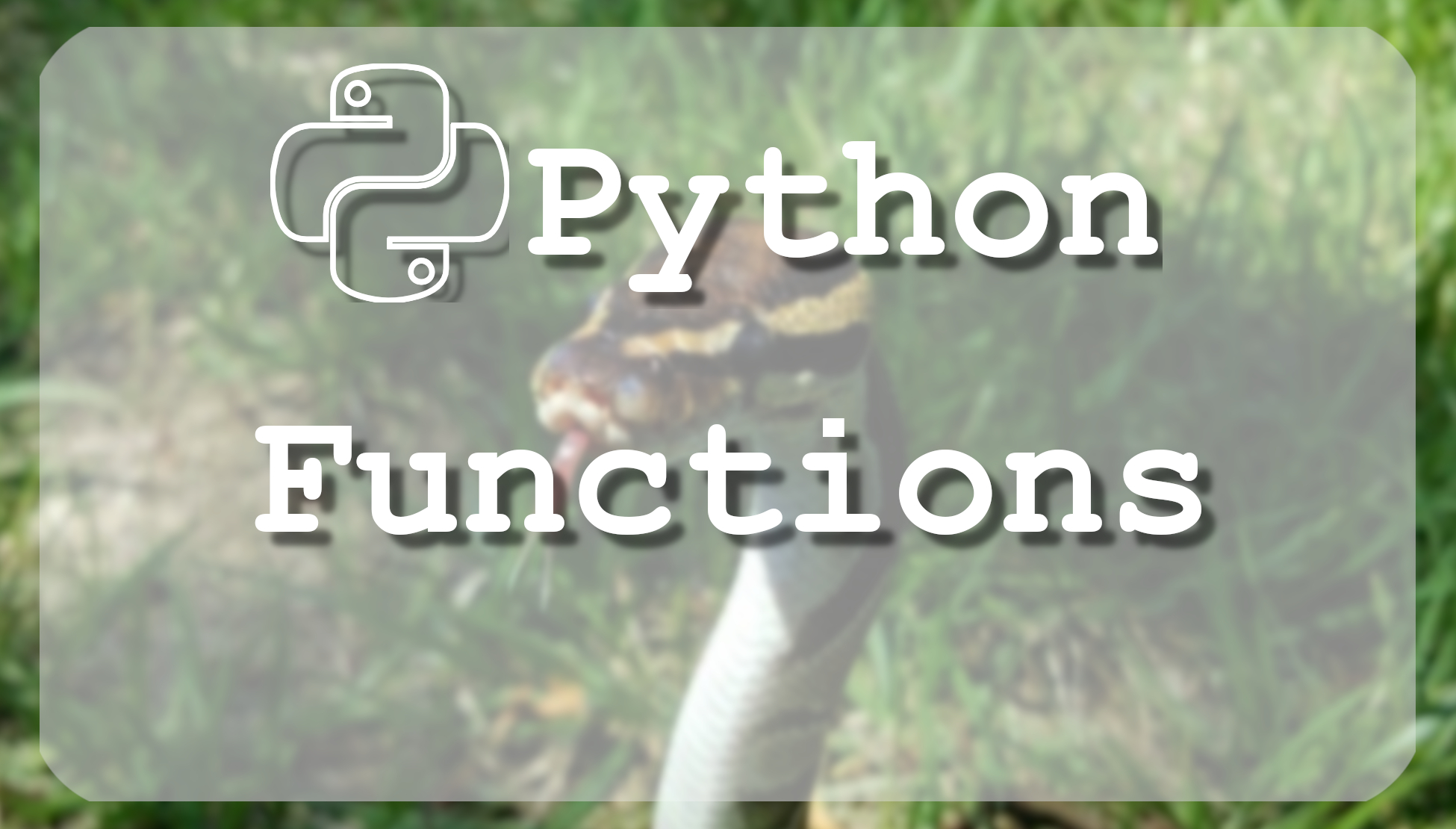# Tutorial - Python 3 basics Python functions

## This tutorial is about Python break, continue and pass control statements, using them we can leave loop, go back or skip certain code.

The Most Advanced Data Science Roadmaps You've Ever Seen! Comes with Thousands of Free Learning Resources and ChatGPT Integration! https://aigents.co/learn/roadmaps/intro

A function is a block of organized, reusable code that is used to perform a single, related action. Functions provide better modularity for applications and a high degree of code reusing. These functions are called user-defined functions and you can create your own functions.

## Defining a Function:

You can define functions to provide the required functionality. Here are simple rules to define Python function:

• Function blocks begin with the keyword def followed by the function name and parentheses ( ( ) ), example: "`def DoSomething():`".
• Any input parameters or arguments should be placed within these parentheses. You can also define parameters inside these parentheses, for example: "`def DoSomething(y, x):`".
• The code block within every function starts with a colon (:) and is indented.
• The statement return [expression] exits a function, optionally passing back an expression to the caller. A return statement with no arguments is the same as a return None.

## Syntax:

``````def functionname(parameters):
function suite
return [expression]``````

By default, function parameters have a positional behavior and you need to inform them in the same order that they were defined. You always must use return with the return value, otherwise, it will return none.

Example:

``````def example():
print("This example will run")
a = 22 + 99
return a

print(example())``````

The above function takes addition as an input parameter and prints it on a standard screen when we call this example() function.

## Function Required Arguments:

Required arguments are the arguments passed to a function in correct positional order. Here, the number of arguments in the function call should match exactly with the function definition.

Example:

``````def printme(str):
print (str) # This prints a passed string into this function
return;

# Now you can call printme function
printme("Hi there")``````

When the above code is executed, it prints: "Hi there".

## Function Keyword Arguments:

Keyword arguments are related to the function calls. When you use keyword arguments in a function call, the caller identifies the arguments by the parameter name.

This allows you to skip arguments or place them out of order because the Python interpreter is able to use the keywords provided to match the values with parameters. You can also make keyword calls to the `printme()` function in the following ways:

``````def printme(str, value):
print (str) # This prints a passed string into this function
print (value) # This prints a passed value into this function
return;

# Now you can call printme function
printme(999, "Hi there")``````

When the above code is executed, it returns the same result as it was with the required arguments: "Hi there" and in a new line number: "999".

## Function Default Arguments:

A default argument is an argument that assumes a default value if the value is not provided in the function call for that argument. The following example gives an idea of default arguments, it prints the default age if it is not passed. Example:

``````def printme(str, value = 77):
print ("string:", str) # This prints a passed string into this function
print ("value:", value) # This prints a passed value into this function
return;

# Now you can call printme function
printme(value = 999, str = "Hi there")
printme(str = "Hi there")``````

When the above code is executed, it prints two results, the first is with custom arguments, second is with one custom and one default argument.

## Function Variable-length Arguments:

Sometimes it may need to process a function for more arguments than you specified while defining the function. These arguments are called variable-length arguments and are not named in the function definition, unlike required and default arguments.

Variable arguments Syntax:

``````def functionname([formal_args,] *var_args):
function suite
return [expression]``````

An asterisk (*) is placed before the variable name that holds the values of all non-keyword variable arguments. This argument remains empty if no additional arguments are specified during the function call.

Example:

``````def printme( args1, *args2 ):
print ("Output is: ")
print (args1)
for var in args2:
print (var)
return;

# Now you can call printinfo function
printme( 10 )
printme( 80, 70, 60, 50 )``````

When the above code is executed, it prints two results, at the first print, we get value 10 and it skips *args2, at the second print, we skip args1 and it prints *args2 values (80, 70, 60, 50).

## Defining anonymous function:

Anonymous functions are called so because they are not declared in the standard manner by using the def keyword. You can use the lambda keyword to create small anonymous functions. Here are simple rules to define Python anonymous function:

• Lambda forms can take any number of arguments but return just one value in the form of an expression. They cannot contain commands or multiple expressions.
• An anonymous function cannot be a direct call to print because lambda requires an expression
• Lambda functions have their own local namespace and cannot access variables other than those in their parameter list and those in the global namespace.
• Although it appears that lambdas are a one-line version of a function, they are not equivalent to inline statements in C or C++, whose purpose is by passing function stack allocation during invocation for performance reasons.

## Syntax:

The syntax of lambda functions contains only a single statement:

``lambda [arg1 [,arg2,.....argN]]:expression``

Following is the example to show how the lambda form of function works:

``````sum = lambda arg1, arg2: arg1 + arg2;
mult = lambda arg1, arg2: arg1 * arg2;

# Now you can call sum and mult as a function
print("Sum value:", sum(5, 10))
print("Sum value:", sum(25, 25))
print("Mult value:", mult(11, 11))``````

In this tutorial, we covered how to define and use various functions in Python.